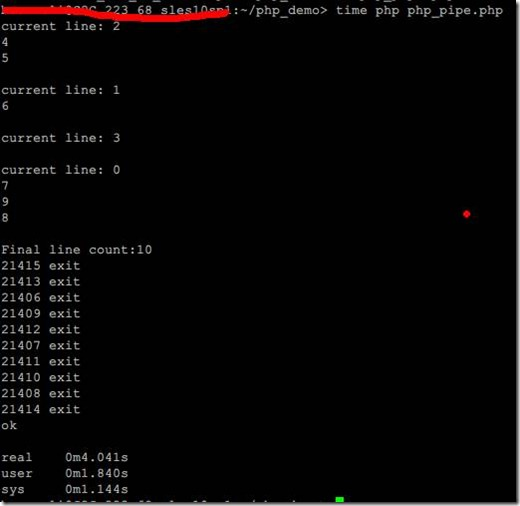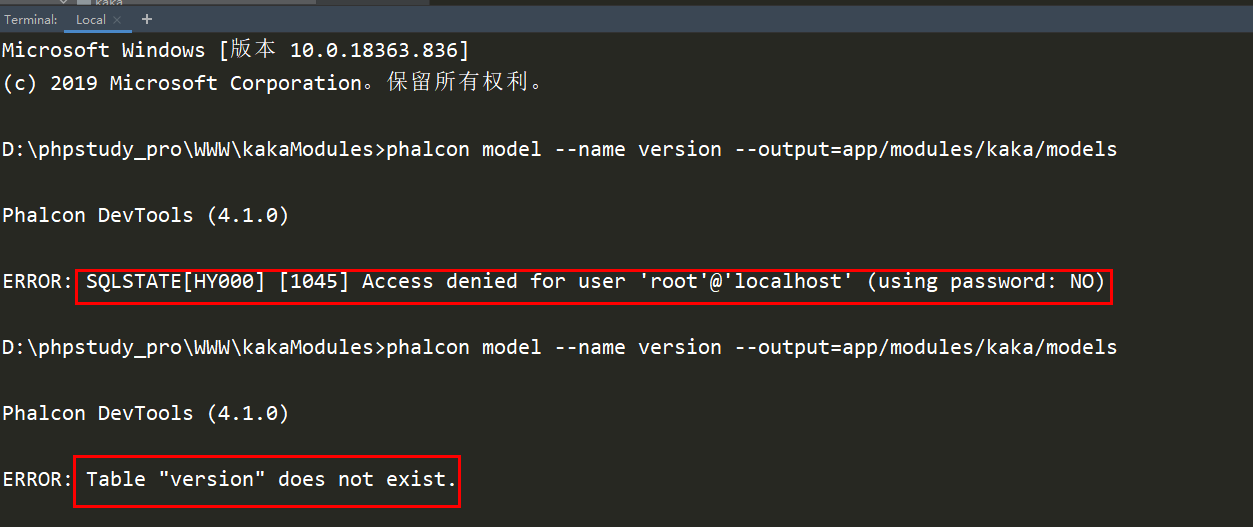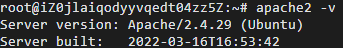# PHP多进程处理并行处理任务实例

+关注继续查看

PHP多进程

 1 2 3 4 5 6 7 8 9 10 11 12 13 14 15 16 17 18 19 20 21 22 23 24 25 26 27 28 29 30 31 32 33 34 35 36 37 38 39 40 41 42 43 44 45 46 47 48 49 50 51 52 53 54 55 56 57 58 59 60 61 62 63 64 65 66 67 68 69 70 71 72 73 74 75 76 77 78 79 80 81 82 83 84 85 /**  * this is a demo for php fork and pipe usage. fork use  * to create child process and pipe is used to sychoroize  * the child process and its main process.  * @author bourneli  * @date: 2012-7-6  */   define("PC", 10); // 进程个数 define("TO", 4); // 超时 define("TS", 4); // 事件跨度，用于模拟任务延时   if (!function_exists('pcntl_fork')) {     die("pcntl_fork not existing"); }   // 创建管道 $sPipePath = "my_pipe.".posix_getpid(); if (!posix_mkfifo($sPipePath, 0666)) {     die("create pipe {$sPipePath} error"); } // 模拟任务并发 for ($i = 0; $i < PC; ++$i ) {     $nPID = pcntl_fork(); // 创建子进程  if ($nPID == 0) {         // 子进程过程         sleep(rand(1,TS)); // 模拟延时         $oW = fopen($sPipePath, 'w');         fwrite($oW, $i."\n"); // 当前任务处理完比，在管道中写入数据         fclose($oW);  exit(0); // 执行完后退出  } } // 父进程 $oR = fopen($sPipePath, 'r'); stream_set_blocking($oR, FALSE); // 将管道设置为非堵塞，用于适应超时机制 $sData = ''; // 存放管道中的数据 $nLine = 0; $nStart = time(); while ($nLine < PC && (time() - $nStart) < TO) {  $sLine = fread($oR, 1024);  if (empty($sLine)) {         continue;        }              echo "current line: {$sLine}\n";  // 用于分析多少任务处理完毕，通过‘\n’标识  foreach(str_split($sLine) as $c) {  if ("\n" == $c) {             ++$nLine;  }  }  $sData .= $sLine; } echo "Final line count:$nLine\n"; fclose($oR); unlink($sPipePath); // 删除管道，已经没有作用了   // 等待子进程执行完毕，避免僵尸进程 $n = 0; while ($n < PC) {     $nStatus = -1;  $nPID = pcntl_wait($nStatus, WNOHANG);  if ($nPID > 0) {         echo "{$nPID} exit\n";  ++$n;     } }   // 验证结果，主要查看结果中是否每个任务都完成了 $arr2 = array(); foreach(explode("\n", $sData) as $i) {// trim all  if (is_numeric(trim($i))) {         array_push($arr2, $i);       } } $arr2 = array_unique($arr2); if ( count($arr2) == PC) {   echo 'ok';  } else {  echo "error count " . count($arr2) . "\n";     var_dump(\$arr2); }

ok，完毕，注释写的比较清除，执行结果如下：Phalcon多模块如何实现连接不同数据库 《Phalcon入坑指南系列 五》（1）
Phalcon多模块如何实现连接不同数据库 《Phalcon入坑指南系列 五》
4 0Serverless 时代，这才是 Web 应用开发正确的打开方式

12 011 043 0Vite 与 Vue Cli 对比 - 尤雨溪: Vite 会取代 vue-cli 吗？

9 011 0
4852

0

OceanBase 入门到实战教程